# Mathematical problems for self assessment

###### APPLICATIONS OF INTEGRATION II

1. Find the length of the curve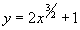between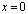and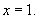[Ans.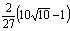]

2. Find the length of the cycloid parameterised by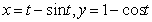between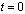and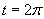.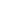[Ans. 8]

3. Find the area of the surface of revolution generated by the curve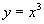when it is rotated about the

x–axis between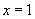and.

[Ans.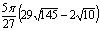.

4. Find the area of the surface of revolution generated by the curve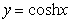when it is rotated about the x-axis betweenand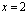.

[Ans.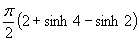]

5. Find the moment of inertia of a thin rod of lengthand mass M about an axis normal to its length and acting through the rod at a distance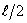from one end.

[Ans.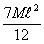]

6. Find the moment of inertia of an annular lamina of mass M with inner radius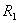and outer radius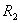about a line L through its centre which is normal to its plane.

[Ans.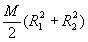]

7. Find the moment of inertia of a thin rectangular plate of mass M, length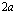and widthabout an axis through its centre of gravity which is normal to its plane.

[Ans.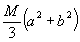]

8. Find the moment of inertia of a thin square plate of mass M and side lengthabout a linewhich lies in the same plane as the plate and which is parallel to, and at a distancefrom, a line L that passes vertically through the centre of gravity of the plate.

[Ans.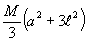]

.

9. Find both the mean and root-mean-square values of the following functions over the given intervals:

(i)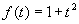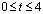,

(ii).

[Ans. (i) mean value = 19/3, r.m.s. value=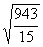, (ii) mean value = 2+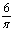, r.m.s. value =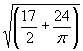].